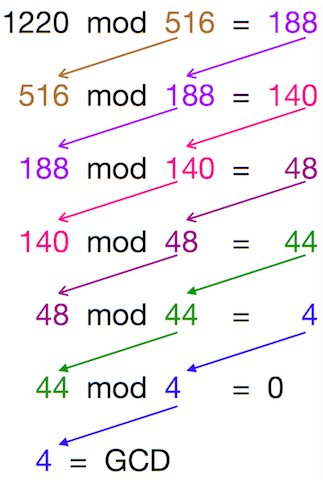# Greatest Common Divisor Euclidean

For this topic you must know about Greatest Common Divisor (GCD) and the MOD operation first.

#### Greatest Common Divisor (GCD)

The GCD of two or more integers is the largest integer that divides each of the integers such that their remainder is zero.

Example-
GCD of 20, 30 = 10 (10 is the largest number which divides 20 and 30 with remainder as 0)
GCD of 42, 120, 285 = 3 (3 is the largest number which divides 42, 120 and 285 with remainder as 0)

#### “mod” Operation

The mod operation gives you the remainder when two positive integers are divided. We write it as follows-
`A mod B = R`

This means, dividing A by B gives you the remainder R, this is different than your division operation which gives you the quotient.

Example-
7 mod 2 = 1 (Dividing 7 by 2 gives the remainder 1)
42 mod 7 = 0 (Dividing 42 by 7 gives the remainder 0)

With the above two concepts understood you will easily understand the Euclidean Algorithm.

### Euclidean Algorithm for Greatest Common Divisor (GCD)

The Euclidean Algorithm finds the GCD of 2 numbers.

You will better understand this Algorithm by seeing it in action. Assuming you want to calculate the GCD of 1220 and 516, lets apply the Euclidean Algorithm-

Assuming you want to calculate the GCD of 1220 and 516, lets apply the Euclidean Algorithm-Pseudo Code of the Algorithm-
Step 1: Let `a, b` be the two numbers
Step 2: `a mod b = R`
Step 3: Let `a = b` and `b = R`
Step 4: Repeat Steps 2 and 3 until `a mod b` is greater than 0
Step 5: GCD = b
Step 6: Finish

JavaScript Code to Perform GCD-

``````function gcd(a, b) {
var R;
while ((a % b) > 0)  {
R = a % b;
a = b;
b = R;
}
return b;
}
``````

JavaScript Code to Perform GCD using Recursion-

``````function gcd(a, b) {
if (b == 0)
return a;
else
return gcd(b, (a % b));
}
``````

C code to perform GCD using recursion

``````int gcd(int a, int b)
{
// Everything divides 0
if (a == 0)
return b;
if (b == 0)
return a;

// base case
if (a == b)
return a;

// a is greater
if (a > b)
return gcd(a-b, b);
return gcd(a, b-a);
}
``````

C++ Code to Perform GCD-

``````int gcd(int a,int b) {
int R;
while ((a % b) > 0)  {
R = a % b;
a = b;
b = R;
}
return b;
}
``````

Python Code to Perform GCD using Recursion

``````def gcd(a, b):
if b == 0:
return a:
else:
return gcd(b, (a % b))
``````

Java Code to Perform GCD using Recursion

``````static int gcd(int a, int b)
{
if(b == 0)
{
return a;
}
return gcd(b, a % b);
}
``````

You can also use the Euclidean Algorithm to find GCD of more than two numbers. Since, GCD is associative, the following operation is valid- `GCD(a,b,c) == GCD(GCD(a,b), c)`

Calculate the GCD of the first two numbers, then find GCD of the result and the next number. Example- `GCD(203,91,77) == GCD(GCD(203,91),77) == GCD(7, 77) == 7`

You can find GCD of `n` numbers in the same way.

### Extended Euclidean algorithm

This is an extension of Euclidean algorithm. It also calculates the coefficients x, y such that

ax+by = gcd(a,b)

x and y are also known as coefficients of Bézout’s identity.

c code for Extended Euclidean algorithm

``````struct Triplet{
int gcd;
int x;
int y;
};
Triplet gcdExtendedEuclid(int a,int b){
//Base Case
if(b==0){
Triplet myAns;
myAns.gcd = a;
myAns.x = 1;
myAns.y = 0;
return myAns;

}
Triplet smallAns = gcdExtendedEuclid(b,a%b);
//Extended euclid says

Triplet myAns;
myAns.gcd = smallAns.gcd;
myAns.x  = smallAns.y;
myAns.y = (smallAns.x - ((a/b)*(smallAns.y)));
return myAns;
}
``````